# Random Sampling and Population Inferences Lesson Plan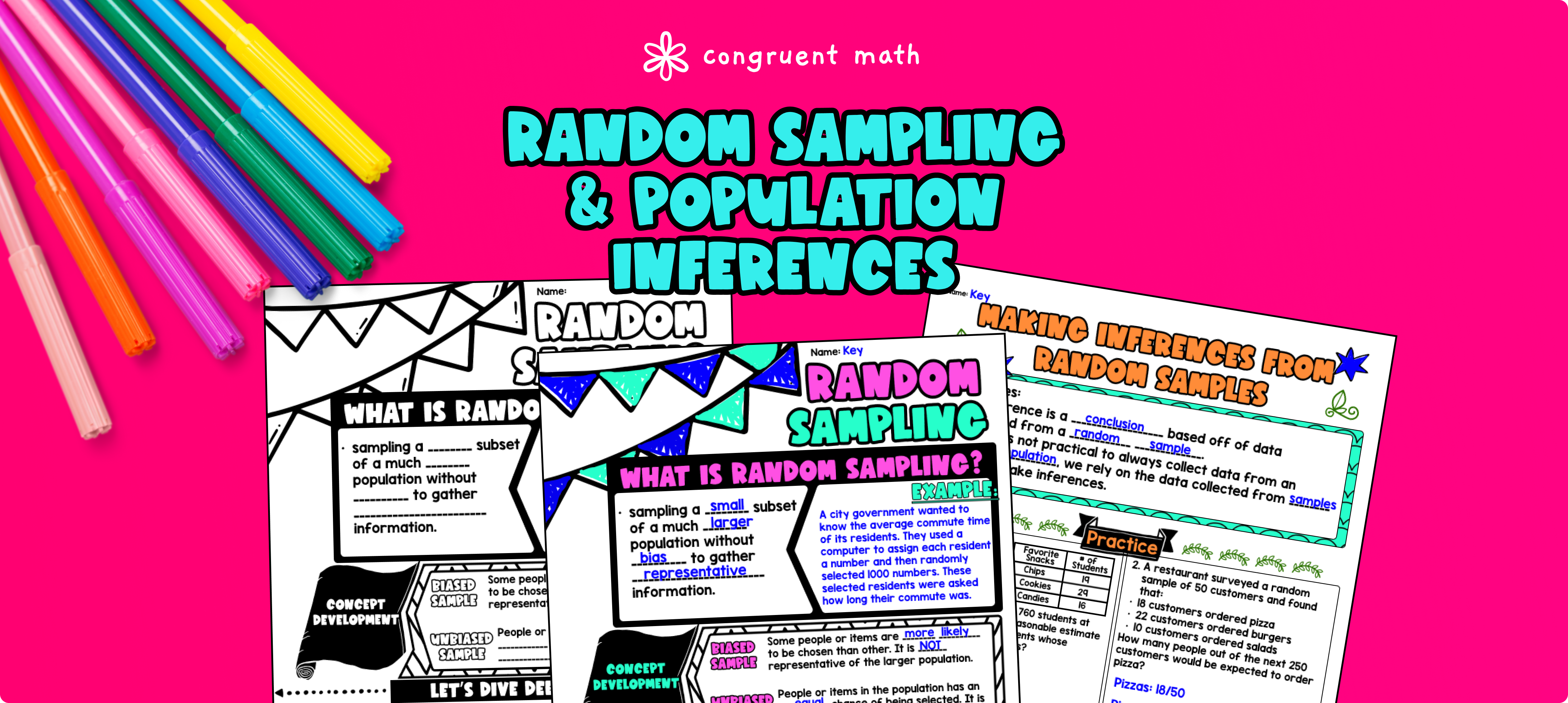Pin This

## Overview

Ever wondered how to teach population inferences and random sampling in an engaging way to your 7th grade students?

In this lesson plan, students will learn about population inferences and how data collected from random samples can be used to draw conclusions about a population. Through artistic and interactive guided notes, students will explore vocabulary terms such as random samples, biased samples, and representative samples. They will also learn how to identify and draw inferences from data collected through random sampling.

To reinforce their understanding, students will engage in word problems practice, a maze worksheet, and a doodle math activity (similar to a color-by-number activity). These exercises will help students practice their skills and solidify their understanding of population inferences and random sampling.

The lesson culminates with a real-life application, where students can read about how random sampling and population inferences are used in market research. This will help students see the practical relevance and real life applications of population inferences and random sampling.

Overall, this lesson plan provides a comprehensive and engaging approach to teaching population inferences and random sampling to 7th grade students.

## Get the Lesson Materials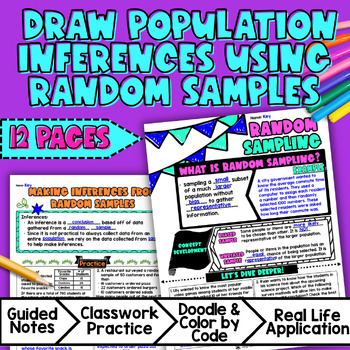\$4.25

## Learning Objectives

After this lesson, students will be able to:

• Define terms related to random sampling, including random sample, population inferences, biased samples, unbiased samples, and representative of the population.

• Explain the concept of a random sample and its importance in making valid inferences about a population.

• Use data from a random sample to draw inferences about a population with an unknown characteristic of interest.

• Generate multiple samples (or simulated samples) of the same size to gauge the variation in estimates or predictions.

• Apply the concepts of random sampling and population inferences to a real-life example of statistical questions in a meaningful way.

## Prerequisites

Before this lesson, students should be familiar with:

• Basic understanding of some statistics and probability concepts, such as data collection, organization, and representation

## Key Vocabulary

• Random samples

• Population inferences

• Biased samples

• Unbiased samples

• Representative of the population

• Data collected

• Statistical questions

## Procedure

### Introduction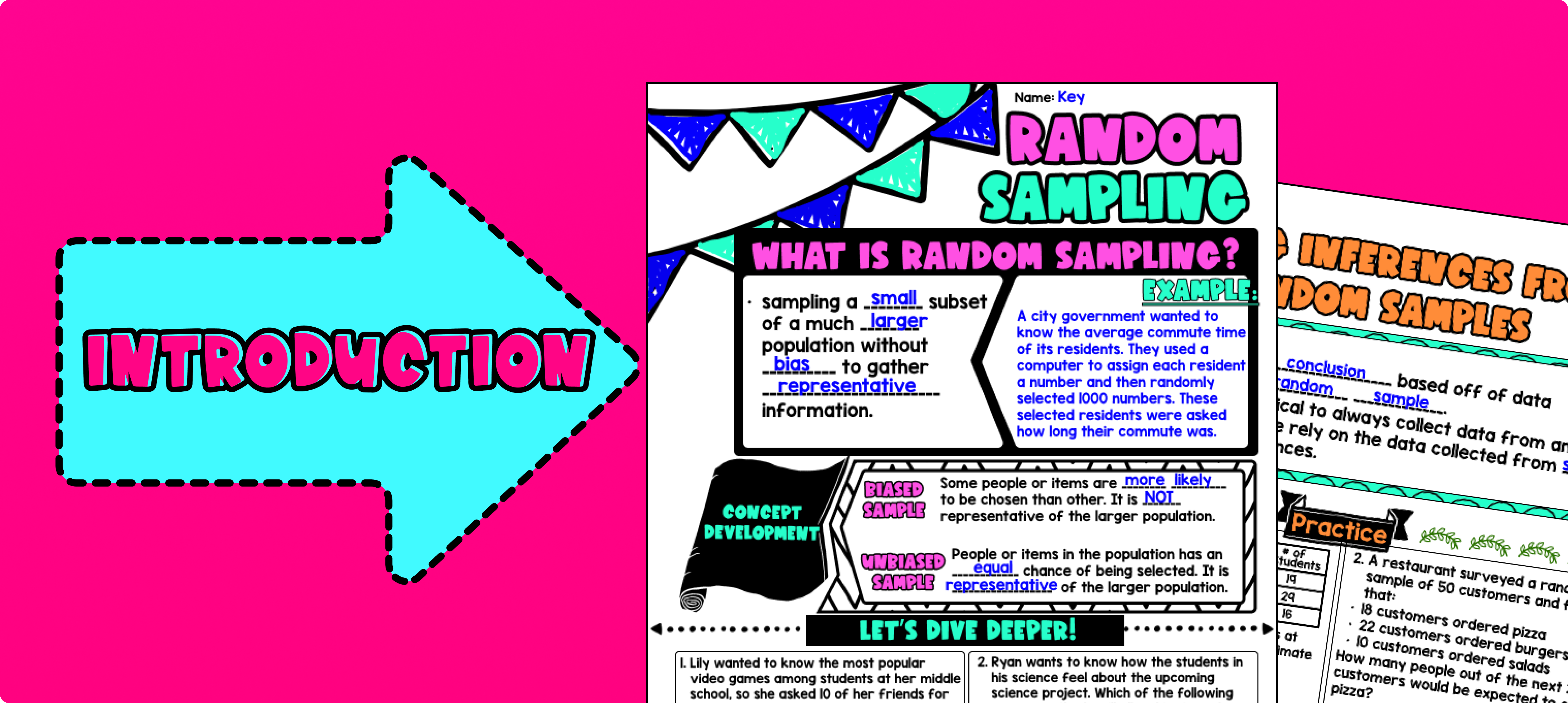Pin This

As a hook, ask students why understanding random sampling and population inferences is important. For example, you could ask them why it is important to have a representative sample when conducting a survey or making predictions about a population. Refer to the last page of the guided notes as well as the FAQs below for ideas.

Use the first page of the guided notes to introduce the concept of random samples and population inferences. Walk through the key vocabulary terms, such as random samples, population inferences, biased samples, unbiased samples, and representative of the population. Explain what each term means and why they are important in statistics.

Use the second page of the guided notes to explain how to draw inferences by using data collected from random samples. Walk through the steps of what inferences are and why they are important. Discuss why random sampling tends to produce representative samples and support valid inferences. Go through the two examples in the guided notes resource. Refer to the FAQ below for a walk through on this, as well as ideas on how to respond to common student questions.

Based on student responses, reteach concepts that students need extra help with. If your class has a wide range of proficiency levels, you can pull out students for reteaching, and have more advanced students begin work on the practice exercises.

### Practice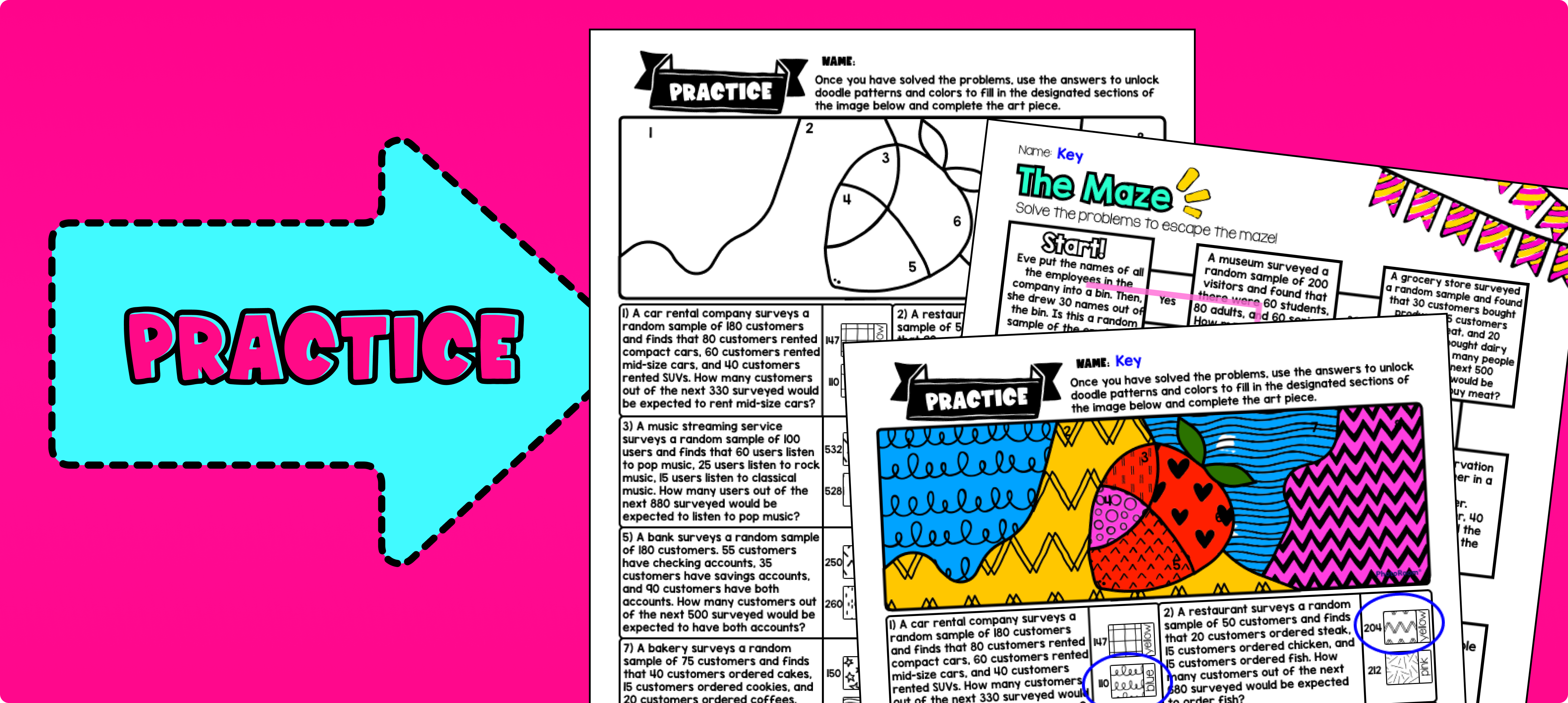Pin This

Have students practice drawing inferences about population using the "You Try" section of the guided notes resource. Walk around to answer student questions.

Fast finishers can dive into the maze activity and doodle math activity. for extra practice. You can assign it as homework for the remainder of the class or complete it as a group activity the next day.

### Real-Life Application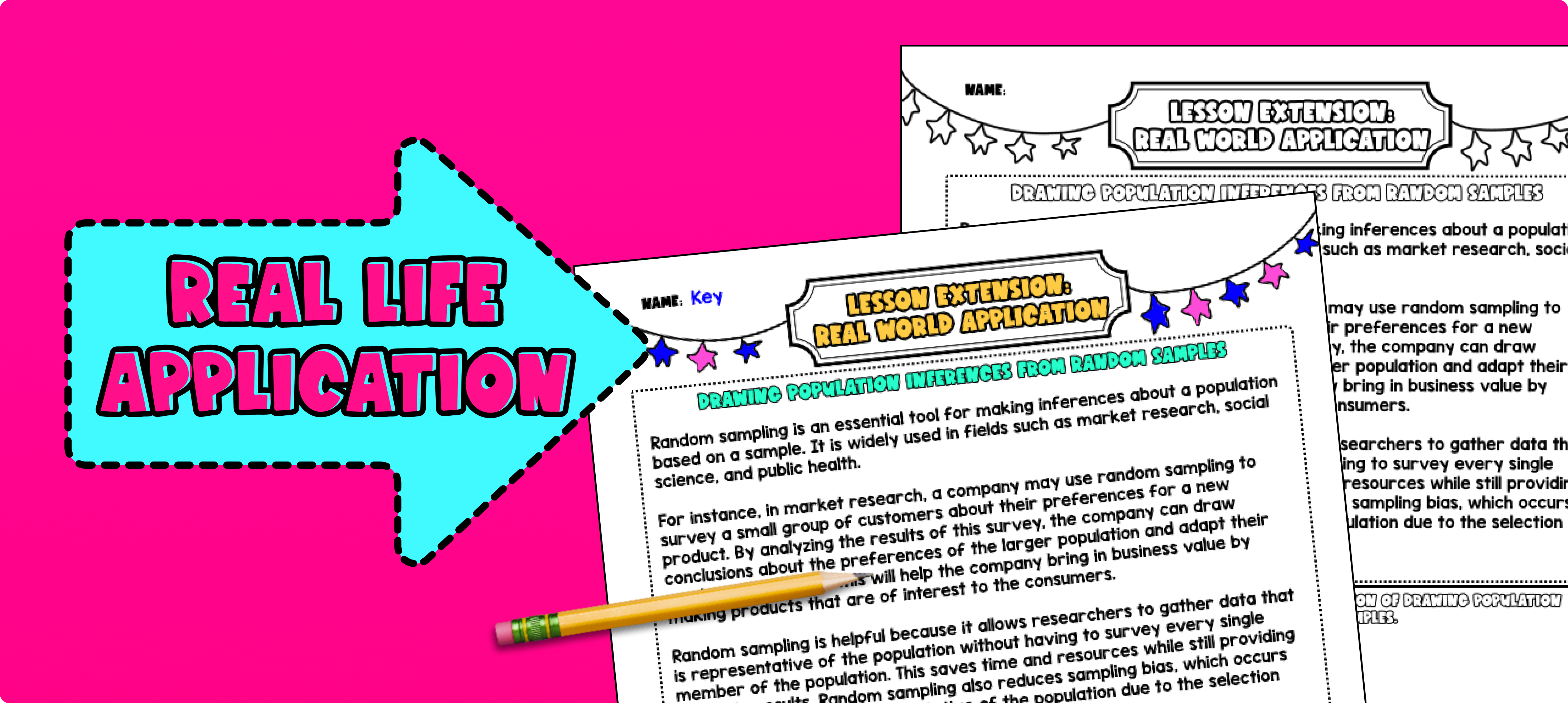Pin This

Bring the class back together, and introduce the concept of real-life statistical questions. Explain to the students that statistics and population inferences play an important role in answering questions and making decisions in various real-life situations. Use the last page of the guided notes resource for discussion prompt and give students a place to reflect on their learning.

One example of a real-life application of population inferences is in market research. Explain to the students that companies often use random sampling and population inferences to make informed decisions about their products or services. For example, a company that produces a new flavor of potato chips may want to know if their target population (consumers in a certain age group or geographic region) will like the new flavor. Instead of surveying every individual in the population, the company can randomly sample a smaller group of individuals and use the data collected to draw inferences about the larger population. This helps the company make decisions about production, marketing, and distribution based on representative data.

Refer to the FAQ section of the lesson plan for more ideas on how to teach real-life applications of random sampling and population inferences.